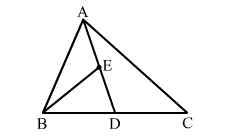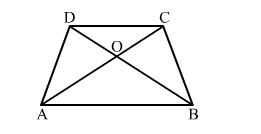# Which of the following is a false statement?

Question:

Which of the following is a false statement?

(a) A median of a triangle divides it into two triangles of equal areas.
(b) The diagonals of a || gm divide it into four triangles of equal areas.

(c) In a $\triangle A B C$, if $E$ is the midpoint of median $A D$, then $\operatorname{ar}(\Delta B E D)=\frac{1}{4} \operatorname{ar}(\Delta A B C)$.(d) In a trap. ABCD, it is given that AB || DC and the diagonals AC and BD intersect at O. Then, ar(∆AOB) = ar(∆COD).Solution:

(d) In a trap. ABCD, it is given that AB || DC and the diagonals AC and BD intersect at O. Then ar(∆AOB) = ar(∆COD).

Consider ∆ADB and ∆ADC, which do not lie on the same base but lie between same parallel lines.
Subtracting $\operatorname{ar}(\triangle A O D)$ from both sides, we get:
$\operatorname{ar}(\triangle A D B)-\operatorname{ar}(\triangle A O D) \neq \operatorname{ar}(\triangle A D C)-\operatorname{ar}(\triangle A O D)$
$\operatorname{Or} \operatorname{ar}(\Delta A O B) \neq \operatorname{ar}(\Delta C O D)$